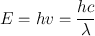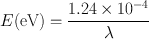# Structure of Matter

(1.1)

where E is energy in ergs , m is the mass in grams, and c is the velocity of light in a vacuum given as 3 × 1010 cm/s. This relationship states that everything around us can be classified as matter or energy.

Radiation is a form of energy in motion through space . It is emitted by one object and absorbed or scattered by another. Radiations are of two types:

1.

Particulate radiations: Examples of these radiations are energetic electrons, protons, neutrons, α-particles, and so forth . They have mass and charge, except neutrons, which are neutral particles. The velocity of their motion depends on their kinetic energy. The particulate radiations originate from radioactive decay , cosmic rays, nuclear reactions, and so forth.

2.

Electromagnetic radiations: These radiations are a form of energy in motion that does not have mass and charge and can propagate as either waves or discrete packets of energy, called the photons or quanta . These radiations travel with the velocity of light. Various examples of electromagnetic radiations include radio waves, visible light, heat waves, γ-radiations, and so forth, and they differ from each other in wavelength and hence in energy. Note that the sound waves are not electromagnetic radiations.

The energy E of an electromagnetic radiation is given by(1.2)

where h is the Planck constant given as 6.625 × 10−27 erg. s/cycle, ν is the frequency in hertz (Hz), defined as 1 cycle per second, λ is the wavelength in centimeters, and c is the velocity of light in vacuum, which is equal to nearly 3 × 1010 cm/s.

The energy of an electromagnetic radiation is given in electron volts (eV) , which is defined as the energy acquired by an electron when accelerated through a potential difference of 1 V. Using 1 eV = 1.602 × 10−12 erg , Eq. (1.2) becomes(1.3)

where λ is given in centimeters. Table 1.1 lists the different electromagnetic radiations along with their frequencies and wavelengths .

Table 1.1

Type

Energy (eV)

Frequency (Hz)

Wavelength (cm)

10−10–10−6

104–108

102–106

Microwave

10−6–10−2

108–1012

10−2–102

Infrared

10−2–1

1012–1014

10−4–10−2

Visible

1–2

1014–1015

10−5–10−4

Ultraviolet

2–100

1015–1016

10−6–0−5

x-Rays and γ-rays

100–107

1016–1021

10−11–10−6

## The Atom

For the purpose of this book, the atom can be considered as the smallest unit in the composition of matter. The atom is composed of a nucleus at the center and one or more electrons orbiting around the nucleus. The nucleus consists of protons and neutrons, collectively called nucleons . The protons are positively charged particles with a mass of 1.00728 amu , and the neutrons are electrically neutral particles with a mass of 1.00867 amu. The electrons are negatively charged particles with a mass of 0.000549 amu. The protons and neutrons are about 1836 times heavier than the electrons but the neutron is heavier than the proton by one electron mass (i.e., by 0.511 MeV). The number of electrons is equal to the number of protons, thus resulting in a neutral atom of an element. The characteristics of these particles are given in Table 1.2 . The size of the atom is about 10−8 cm (called the angstrom, Å), whereas the nucleus has the size of 10−13 cm (termed the Fermi, F). The density of the nucleus is of the order of 1014 g/cm3. The electronic arrangement determines the chemical properties of an element, whereas the nuclear structure dictates the stability and radioactive transformation of the atom.

Table 1.2
Characteristics of electrons and nucleons.

Particle

Charge

Mass (amu)a

Mass (kg)

Mass (MeV)b

Electron

− 1

0.000549

0.9108 × 10−30

0.511

Proton

+ 1

1.00728

1.6721 × 10−27

938.78

Neutron

0

1.00867

1.6744 × 10−27

939.07

aamu = 1 atomic mass unit = 1.66 × 10−27 kg = 1/12 of the mass of 12C.

b1 atomic mass unit = 931 MeV.

### Electronic Structure of the Atom

Several theories have been put forward to describe the electronic structure of the atom, among which the theory of Niels Bohr, proposed in 1913, is the most plausible one and still holds today. The Bohr’s atomic theory states that electrons rotate around the nucleus in discrete energy shells that are stationary and arranged in increasing order of energy. These shells are designated as the K shell, L shell, M shell, N shell, and so forth. When an electron jumps from the upper shell to the lower shell, the difference in energy between the two shells appears as electromagnetic radiations or photons. When an electron is raised from the lower shell to the upper shell, the energy difference is absorbed and must be supplied for the process to occur.

The detailed description of the Bohr’s atomic structure is provided by the quantum theory in physics. According to this theory, each shell is designated by a quantum number n , called the principal quantum number, and denoted by integers, for example, 1 for the K shell, 2 for the L shell, 3 for the M shell, 4 for the N shell, and 5 for the O shell. Each energy shell is subdivided into subshells or orbitals, which are designated as s, p, d, f, and so on. For a principal quantum number n, there are n orbitals in a given shell. These orbitals are assigned the azimuthal quantum numbers, l, which represent the electron’s angular momentum and can assume numerical values of l = 0,1,2… n−1. Thus for the s orbital, l = 0; the p orbital, l = 1; the d orbital, l = 2; the f orbital, l = 3; and so forth. According to this description, the K shell has one orbital, designated as 1s, the L shell has two orbitals, designated as 2s and 2p, and so forth. The orientation of the electron’s magnetic moment in a magnetic field is described by the magnetic quantum number, m. The values of m can be m = − l,  −  (l − 1),…, 0,… (l − 1), l. Each electron rotates about its own axis clockwise or anticlockwise, and the spin quantum number, s (s = − 1/2 or + 1/2) is assigned to each electron to specify this rotation.

The electron configuration of the atoms of different elements is governed by the following rules:

1.

No two electrons can have the same values for all four quantum numbers in a given atom.

2.

The orbital of the lowest energy will be filled in first, followed by the next higher energy orbital. The relative energies of the orbitals are 1s < 2s < 2p < 3s < 3p < 4s < 3d < 4p < 5s < 4d < 5p < 6s < 4f < 5d < 6p < 7s. This order of energy is valid for lighter elements and is somewhat different in heavier elements.